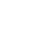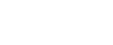## QUESTIONS

1. You are provided with the following.
• A millammeter.
• A voltmeter.
• A wire mounted on a mm scale.
• A switch.
• A long wire with a crocodile clip at one and (crocodile clip to be used as a slider or jockey).
• A new dry cell (size D) and a cell holder.
• A micrometer screw gauge (may be shared).
• 5 connecting wires, two with crocodile clips at the end.

Proceed as follows:

1. Measure the diameter, d of the mounted at three different points.
Average diameter d = _____________________mm (1mk)
2. Set up the apparatus as shown in the circuit diagram in the figure below.3. Close the switch and tap the mounted wire with the crocodile clip as shown in the circuit. Ensure that both meters show positive deflection. Open the switch.
4. Tap the wire at L = 20cm. Close the switch read and record in the time provided the milliammeter and voltmeter reading.
5. Repeat the procedure in (c) for other values of L, shown in the table below and complete the table. (8mks)
 L(cm) L(m) V (Volts) I   MA Amps R = V       I 20 30 40 50 60 80
6.
1. Plot the graph of R (Y-axis) against L(m). (5mks)2. Determine the slope of the graph. (3mks)
3. Given that R = PL/A were A is the cross-sectional area of the wire and P is a constant for the material of the wire, determine the value of the constant P. (3mks)

2. You are provided with the following:

• A marble with a piece of thread attached.
• Two wooden blocks.
• Clamp, boss and retort stand.
• Meter rule.
• metre rule attached to a wooden block.
• Cello tape (2 pieces of about 10cm long)
• Stop watch.

Proceed as follows:

1. Fix the thread between the two wooden blocks and fasten the clamp.
2. Adjust the thread so that the length L shown in figure 1 is 50.0cm.
Fix the metre rule horizontally to the bench using the cello tape provided.
3. Adjust the clamp so that the marble is next to the end of the metre rule as shown.1. Displace the marble by a horizontal distance X = 20cm and measure the corresponding vertical.
Displacement h = ____________________________ cm (1mk)
2. Repeat the experiment to find h for each of the following values in the table.
(Complete the table). (2mks)
 c(cm) h(cm) cÂ²(cmÂ²) cÂ²/h(cm) 20 200 25 625 30 900 35 1225 40 1600 45 2025
3. Plot the graph of(y-axis against h. Draw the best line through the points.(5mks)4. Determine the slope of the graph. (2mks)
5. From the graph, find the value ofwhen h = 0. (1mk)
4. Raise the clamp slightly without changing the length L so that the marble is free to swing. Determine the period, T, for one complete oscillation by timing ten oscillations.
Time for 10 oscillation = ____________________________________(1mk)
Period T = _______________________________________________(1mk)
5. Calculate the value of P from the following equation.where g = 9.8msËÂ² (2mks)You are provided with the following apparatus:

• Candle
• Lens
• Lens holder
• Metre rule
• Cross wire
• Screen
• Vernier calipers

Proceed as follows:

1.  Arrange the apparatus as shown in the figure 2 below.Place the cross-wire before the lens so that U = 28cm. The lit candle should be placed close to the cross-wire.
2. Adjust the position of the screen until a sharp image is cast on the screen.
3. Measure and record the value of image distance, V, in the table.
4. Repeat the same procedure for the other values in the table.

Table 2

 U(cm) V(cm) M = V       U 30 36

5. Given that the focal length f of the lens satisfies the equation f =    V     determine average value of the focal length, f. (3mks)                                                                                                            1 + M

## CONFIDENTIAL

INSTRUCTIONS TO SCHOOL:

1. The information contained in this paper is to enable the head of school and the teacher in charge of Physics to make adequate preparations for the Joint Physics
Practical Examination. NO ONE ELSE should have access to this paper or acquire knowledge of its contents. Great care MUST be taken to ensure that the information herein does not reach the candidates either directly or indirectly.
2. The apparatus required by each candidate for the Physics Practical Examination are set out on page 2 and 3. It is expected that ordinary apparatus of Physics laboratory will be available.
3. The Physics teacher should note that it is his/her responsibility to ensure that each apparatus require for this examination requires with the specifications on page 2 and 3.
4. The question paper will not be opened in advance.
5. The Physics teacher is not expected to perform the experiments.

NB: Any use of apparatus other than the ones specified may leads to candiates being penalized.

QUESTION 1
Each candidate will require the following.

• A milliammeter.
• A voltmeter (0 â 3V) or (0 â 5V).
• A wire mounted on a mm scale (Nichrome wire SWG 32)
• A switch.
• A long wire with a crocodile clip at one end (crocodile clip to be used as a slider or jockey).
• A micrometer screw gauge (may be shared).
• 5 connecting wires, two with crocodile clips at the end.
• One new dry cell size D.
• Cell holder.

QUESTION 2

• A metallic marble (bob) with hook.
• Two wooden blocks measuring 1cm Ã 3cm Ã 4cm.
• Clamp, boss and retort stand.
• Meter rule.
• Stop watch.
• Cello tape (2 pieces of about 10cm long).
• 1/2 metre rule attached to a wooden block of (5cm Ã 5cm Ã 5cm) as shown using cello tape/plastine.• Candle.
• Lens of focal length 20cm (convex).
• Lens holder.
• Cardboard of 2cm Ã 20cm with a hole of diameter approximately 4cm at the centre (cross wire placed at the hole)
• Screen (white).
• Vernier calipers (to be shared).
• Match box (to be shared).
• Metre rule.

NB: The cardboard and screen to be supported on wooden block to enable them stand vertically upright.

## MARKING SCHEME

1.
1. Evaluation of d (1mk)
d = 0.42mm = 4.2 Ã 10-4
2.
3.
4.Each correct entry of V columns (Â½mk)
Each correct entry of MA column (Â½mk)
At least 3 correctly corrected Amps (1mk)
At least 3 correctly evaluated vales of R (1mk)
Error Voltmeter Â± 0.01
Milliameter Â± 0.01
5.
1.
2. Suitable scale (1mk)
Labeling units indicated (1mk)
Plotting 5 - 6 correct points (2mks)
or 3 â 4 correct points (1mk)
Suitable straight line (1mk)
See graph paper attached.ÎL(M)
= 5000 - 1000
0.8 - 0.2
- substitution
= 4000
0.6
4. R = Pl
A
Comparing with
y = MX + C
A
= p   = slope Ã A
= 6666 Ã 22 Ã (2.1 Ã 10-4)2
7
2.
1.
1. h = 4.0 Â± 0.5 (1mk)
2.
 (ii) h(cm) Ï2/h(cm) 4.0 100.00 cÂ²h correct (2mks) 6.0 104.18 Ay 5 h correct (1mk) 9.5 94.74 14.0 87.50 20.0 80.00 29.0 69.83
3. slope = Îx2h = 65 - 92.5 = -27.5  (1mk)
Îh         35 - 10        25
= -1.1 (1mk)
4.
5. x2 = 104.5 Â± 1 (1mk)
h
2.
1. Time for oscillations = 27.66 sec. Â±  1 sec. (1mk)
Period T = 1.383
2.2(b)U(cm) V(cm) Â± 2 M = V/U 30 58 1.933 36 44 1.222 (1mk)

F =     V
M + 1
F1 =      58       = 19.775
1.933 + 1
F2 =     44      =  19.802
1.222 + 1
F = F1 + F2
2
19.775 + 19.802 = 19.788
2## CONFIDENTIAL

1. Constantan wire SWG 26 mounted on a metre rule
2.  Ammeter ( 0 â 1)A
3. Voltmeter ( 0 â2.5)V
4. A jockey
5. 6 connecting wires with crocodile clips
6.  A switch
7.  A new dry cell and a cell holder
8. Micrometer screw gauge to be shared

QUESTION 2

1. copper wire of length = 150cm

Specification

1.
1. Diameter = 0.7mm
2. Size = 22
3. Length = 150cm
2. 50g mass
3. A metre rule
4. two pieces of wood
5. Test tube
6. Retort stand, boss and clamp

N/B
The copper wire is found in any electrical shop. It is suitable for making a simple spiral spring. The wire is coated and it is flexible. The sample of the same can be obtained in the following electrical shops.